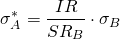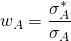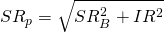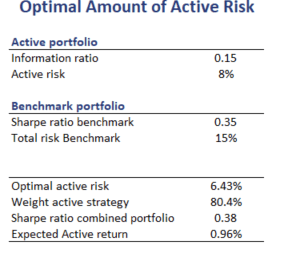# Optimal amount of active risk

The optimal amount of risk tells you the level of active risk that maximises the portfolio Sharpe ratio. On this page, we discuss how an investor can easily calculate the optimal amount of risk. To do so, we need the Sharpe ratio of the passive portfolio, the volatility of the passive portfolio, as well as the information ratio of the active portfolio.

## Optimal amount of active risk formula

For an unconstrained active portfolio, the optimal amount of risk is the level of active risk that maximises the overall Sharpe ratio. By that we mean that, if we allocate that calculated proportion of the portfolio to the active strategy, the overall Sharpe ratio will be the highest. The formula to calculate the optimal amount of risk is the followingwhere IR is the information ratio of the active portfolio, b is the Sharpe ratio of the benchmark and sigma B is the volatility of the benchmark. Once we have the optimal amount of active risk, we still need to determine the amount we should invest in the active portfolio and the amount in the benchmark portfolio. To calculate these, we use the formulawhere sigma A* and A are the optimal amount of active risk and the total active risk of the active portfolio. We can also calculate the Sharpe ratio of the combined portfolio as follows## Optimal amount of active risk example

Finally, let’s turn to an example of what active risk should a portfolio should take given a certain information ratio. The following table implements the above formulae using a numerical example. The spreadsheet can be downloaded below.## Summary

We discussed the calculation of the amount of active risk an investors should take. This is the amount of risk that maximizes the Sharpe ratio of the overall portfolio. This method can help investors allocate between an active portfolio (e.g. a mutual fund) with a certain information ratio and the benchmark portfolio. The latter is, of course, cheaper since the management fee is considerably lower.